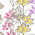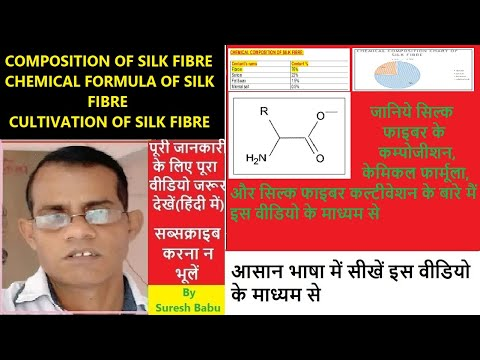## Saturday, April 27, 2019

### FABRIC COSTING

FABRIC COSTING:

The fabric costing is a very important aspect for any fabric manufacturer, merchandiser, textile exporter and Weaving technician. If a person knows, how to calculate the weaving cost of a fabric? He can finalize the order on the spot. An exporter can make a correct decision during purchase of fabric. A sourcing manager can get competitive price of fabric if he knows the method of fabric costing calculation.

There are following types of expenses occur in fabric manufacturing.

1- yarn cost.

2- Sizing cost.

3- Weaving cost.

1-Yarn cost:

We know that the fabric is made of warp and weft. Here the warp and weft weight is calculated separately. Before starting the fabric costing, we should know about fabric parameters. You should read my old blog on FABRIC PARAMETERS AND FABRIC ANALYSIS first to know about all details about fabric. The all fabric parameters are tested precisely in in-house or outside laboratory. Suppose that we receive following fabric parameters which are required to calculate the fabric cost:

1- EPI = 100

2- PPI = 80

3- Warp count =  40 s cotton

4- Weft count = 40 s cotton

5- Fabric width=120 inches

6- Warp crimp = 11 ℅

7- Weft crimp = 4℅

8- Waste percentage= 3℅

9- Warp yarn rate = Rs 250 per kg.

10- Weft yarn rate = Rs. 250 per kg

11 -Sizing cost = Rs. 20 per kg of warp

Warp weight:

It is determined by using following formula:

Weft weight:

It is calculated by using below formula:

Please not the yarn waste 3℅ is for rapier looms. It may be vary according to type of loom.

Sizing cost :

It is calculated by using following formula:
After finding of warp and weft weight, we can calculate the yarn cost only.
Sizing cost is the next step. Sizing cost  has following factors:

1 – chemical cost

2 – power cost

3 – men power cost

4 – depreciation

5 - maintenance cost

Today sizing cost comes around RS. 20 per kg of warp for 40 s Ne yarn. It may be vary according to count. Sizing cost is low for coarse yarn and high for fine yarn.

We can calculate the sizing cost of warp of one meter of fabric by using following formula:

Weaving cost :

Now we will discuss about weaving cost. Following factors are involved in weaving cost.

1. Power cost

2. Men power cost

3. Maintenance cost

4. Depreciation cost.

These costs are calculated for one meter of the fabric. Today’s weaving cost for this construction comes around Rs. 20 per meter.
When we find weaving cost per meter of fabric , we also calculate weaving cost per pick
What is this weaving cost per pick.
It is the cost incurring for one meter fabric when the picks per inch is 1.
It is calculated as below:
Weaving cost sheet :

Now we have found all cost factors. The complete weaving cost sheet is given below :

You may also interested in following articles:

1.plz tell in hindi

2.This is the best way to explain weaving costing thanks sir

3.Very Very Thanks Sir,

4.This post was very informative. Request you to make a Post on spinning and processing calculations too.

5.That's easy method thanks sir

6.Very good information sir

7.thanks for this information

8.EXAMPLE
WE PAID GST ON POLYESTER YARN IS 12%
AND WE CHARGE GST ON POLYESTER FABRIC IS 5%, THAN HOW TO MANAGE GST IN OUR FABRIC COSTING.

9.10.This comment has been removed by a blog administrator.

11.How to find epi and ppi

1.Read my blog FABRIC ANALYSIS for EPI and PPI

12.Pls give me cost of 60*60 96*90
50 inch
Yarn rate for example 500 rupees kg.

13.This is jocking. If i know every average rate such as yarn cost, sizing cost, weaving cost it’s very easy to find fabric selling cost. We want to know about how to costing sizing cost, weaving cost broadly which factors are involve such as power cost, man power cost, maintenance cost, depreciation cost. If u know about this please share...

## Featured Post

### COMPOSITION OF SILK FIBRE l CHEMICAL FORMULA OF SILK FIBRE l CULTIVATION...